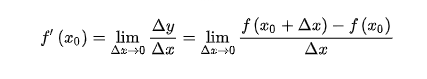• 今天我们来讲一下，使用python求自定义函数求倒数的程序 对于这个程序，我们的原理来自于导数的计算公式如下： 那么根据如上公式，我们得到代码如下： #求函数导数 import os import numpy as np #定义一个函数y=...
求自定义函数的导数
今天我们来讲一下，使用python求自定义函数求倒数的程序
对于这个程序，我们的原理来自于导数的计算公式如下：那么根据如上公式，我们得到代码如下：
#求函数的导数
import os
import numpy as np
#定义一个函数y=x**2

def  y(x):
return x**2

def  derivative(x0,deltaX):#x0为所求点横坐标,deltaX为偏移量
return (y(x0+deltaX)-y(x0))/deltaX
der=derivative(2,0.1**10)
print("对于y=x**2 在 2处的导数为：", der)
os.system("pause")

最后，我们可以自行修改y()函数，来定义我们想要求解函数的导数
注：该结果求解的导数是近似解


展开全文算法
• 当然，在另一个答案中给出的subs选项可用于计算某个数的导数，但如果要绘制导数，则不起作用。有一种方法可以解决这个问题：lambdify，如下所述。使用lambdify将所有sympy函数（可以区分但不能计算）转换为它们的...
这个问题的答案很简单。当然，在另一个答案中给出的subs选项可用于计算某个数的导数，但如果要绘制导数，则不起作用。有一种方法可以解决这个问题：lambdify，如下所述。使用lambdify将所有sympy函数（可以区分但不能计算）转换为它们的numpy对应函数（可以计算、绘制等，但不能区分）。例如，sym.sin（x）将替换为np.sin（x）。其思想是：使用symphy函数定义函数，根据需要进行区分，然后定义一个新的函数，它是原始函数的lambdified版本。在下面的代码中，sym.lambdify接受以下输入：sym.lambdify(variable, function(variable), "numpy")第三个输入“numpy”是用numpy对应函数替换sympy函数的内容。例如：def f(x):return sym.cos(x)def fprime(x):return sym.diff(f(x),x)fprimeLambdified = sym.lambdify(x,f(x),"numpy")然后函数fprime(x)返回-sym.sin(x)，函数fprimeLambdified(x)返回-np.sin(x)。我们现在可以在特定的输入值处“调用”或“求值”，而不能“调用”或“求值”，因为前者由numpy表达式和后者的sympy表达式组成。换句话说，输入fprimelambdified(math.pi)是有意义的，这将返回一个输出，而fprime(math.pi)将返回一个错误。下面是在多个变量中使用sym.lambdify的示例。import sympy as symimport mathdef f(x,y):return x**2 + x*y**2x, y = sym.symbols('x y')def fprime(x,y):return sym.diff(f(x,y),x)print(fprime(x,y)) #This works.DerivativeOfF = sym.lambdify((x,y),fprime(x,y),"numpy")print(DerivativeOfF(1,1))
展开全文• 394.0388.0389.0388.0388.0392.0393.0395.0395.0394.0394.0390.0392.0按照之前所了解的，对离散值求导其实就是差分，例如第i点的导数(差分)为：即在一个宽度为2m+1的窗口内通过计算前后m个值加权后的和得到。...
有一组4096长度的数据，需要找到一阶导数从正到负的点，和三阶导数从负到正的点，截取了一小段。394.0388.0389.0388.0388.0392.0393.0395.0395.0394.0394.0390.0392.0按照之前所了解的，对离散值求导其实就是求差分，例如第i点的导数(差分)为：即在一个宽度为2m+1的窗口内通过计算前后m个值加权后的和得到。但是在实际使用过程中效果不是很好。于是想到了同样在一个宽度为2k+1的窗口内，将这2k+1个点拟合成一个函数，然后求导就可以得到任意阶数的导数值。首先是函数拟合，使用from scipy.optimize import leastsq即最小二乘拟合from scipy.optimize import leastsqclass search(object):def __init__(self, filename):self.filename = filenamedef func(self, x, p):f = np.poly1d(p)return f(x)def residuals(self, p, x, y, reg):regularization = 0.1 # 正则化系数lambdaret = y - self.func(x, p)if reg == 1:ret = np.append(ret, np.sqrt(regularization) * p)return retdef LeastSquare(self, data, k=100, order=4, reg=1, show=1): # k为求导窗口宽度,order为多项式阶数,reg为是否正则化l = self.lenstep = 2 * k + 1p =  * orderfor i in range(0, l, step):if i + step < l:y = data[i:i + step]x = np.arange(i, i + step)else:y = data[i:]x = np.arange(i, l)try:r = leastsq(self.residuals, p, args=(x, y, reg))except:print("Error - curve_fit failed")fun = np.poly1d(r) # 返回拟合方程系数df_1 = np.poly1d.deriv(fun) # 求得导函数df_2 = np.poly1d.deriv(df_1)df_3 = np.poly1d.deriv(df_2)df_value = df_1(x)df3_value = df_3(x)fun = np.poly1d(r),fun返回的是一个 polynomial class，具体使用可以见官方文档numpy.poly1dpolynomial对象可以使用deriv方法求导数，求得的依然是 polynomial对象。 df_value = df_1(x)所得到的就是x这个几个点求得的导数值。看似大功告成，但是求导的结果并不是很好，如下图，实际最高点在100左右，但是拟合出来的曲线最高点在120左右，而原因在于使用多项式拟合很难准确拟合曲线。于是想用高斯函数来实现对曲线的拟合，在matlab中试了下，三阶高斯拟合可以很好的拟合曲线，但是numpy以及sicpy中没有找到类似poly1d这种对象，虽然可以自己定义高斯函数，如下def gaussian(self, x, *param):fun = param*np.exp(-np.power(x - param, 2.) / (2 * np.power(param,    2.)))+param*np.exp(-np.power(x - param, 2.) / (2 * np.power(param, 2.)))return fun但是，在通过最小二乘拟合得到函数参数后只能得到拟合后的点，无法直接求导数..所以并不适合。所以还是只能回到多项式拟合，如果4阶多项式不能表征的话，更高阶的呢总体来说，效果还是可以接受的。如果下阶段找到好的高斯函数拟合方法，会继续更新。以上这篇Python求离散序列导数的示例就是小编分享给大家的全部内容了，希望能给大家一个参考，也希望大家多多支持脚本之家。
展开全文• 您有四个选项有限差异不需要外部工具，但容易出现数字错误，如果您处于多...看看autowrap或lambdify函数或查看Jensen’s blogpost about a similar question。自动衍生品非常酷，不容易出现数字错误，但需要一些额...
您有四个选项有限差异不需要外部工具，但容易出现数字错误，如果您处于多变量情况，可能需要一段时间。如果你的问题很简单，符号分化是理想的。符号方法现在变得相当健壮。 SymPy是一个优秀的项目，它与NumPy很好地集成。看看autowrap或lambdify函数或查看Jensen’s blogpost about a similar question。自动衍生品非常酷，不容易出现数字错误，但需要一些额外的库(google为这个，有几个好的选择)。这是最强大的，但也是最复杂/难以设置的选择。如果你很好地限制自己numpy语法，那么Theano可能是一个不错的选择。这里是一个使用SymPy的例子In : from sympy import *In : import numpy as npIn : x = Symbol('x')In : y = x**2 + 1In : yprime = y.diff(x)In : yprimeOut: 2⋅xIn : f = lambdify(x, yprime, 'numpy')In : f(np.ones(5))Out: [ 2.  2.  2.  2.  2.]
展开全文• diff(f,x)diff(f, x)diff(f,x)求导数可引入求微分方程 sympy 求微分方程.(点击可跳转) 1.一阶导数 基本格式 print(diff(f, x)) # f为所求导函数，x为对x进行求导 例：求该函数的导数 f(x)=cos(x)f(x)=cos(x)f(x)=...
• 在matlab中求解的代码：>> syms w;F(w)=8*exp(-j*5*w)/(16+w^2);diff(F(w),w,2)ans =- (200*exp(-w*5*i))/(w^2 + 16) - (16*exp(-w*5*i))/(w^2 + 16)^2 + (64*w^2*exp(-w*5*i))/(w^2 + 16)^3 + (w*exp(-w*5*i)...
• 按照之前所了解的，对离散值求导其实就是差分，例如第i点的导数（差分）为： 即在一个宽度为2m+1的窗口内通过计算前后m个值加权后的和得到。但是在实际使用过程中效果不是很好。于是想到了同样在一个宽度为2k+1的...
• 请注意，如果有0个符号（例如diff（f（x），x，0）），那么即使评估= False，结果也将是该函数（零导数）。 Examples ======== >>> from sympy import sin, cos, Function, diff >>> from sympy.abc import x, y >>...
• 前面研究了极值存在的条件，不知你们是怎么完成的，我们接着研究这道题，【解析】函数有极值，则导数 有变号零点.转化为方程 有解问题，观察函数 与 有交点,由直线和 都过定点 ，只要他们不在相切，则必定相交.而...
• 高考中的导数：函数不等式的整数解问题的解法 高考中的导数：函数的分界线 杨志明：高考中的定积分 杨志明：求导数的常用方法 杨志明：导数的定义及几何意义 导数的四则运算法则及其应用 杨志明：求定积分的常用方法...
• 假设您有两个变量：x = tf.Variable(np.random.random_sample(), dtype=tf.float32)y = tf.Variable(np.random.random_sample(), dtype=tf.float32)以及使用这两个变量定义的函数：f = tf.pow(x, cons(2)) + cons(2)...
• 2019独角兽企业重金招聘Python工程师标准>>> ...
• 假设有两个变量：x = tf.Variable(np.random.random_sample(), dtype=tf.float32)y = tf.Variable(np.random.random_sample(), dtype=tf.float32)以及使用这两个变量定义的函数：f = tf.pow(x, cons(2)) + cons(2) *...
• 展开全部设参数方程 x(t), y(t)，则二阶导数：一阶...连续函数的一阶导数就是相应的切线斜率。一阶导数大于0，则递增；一阶导数小于0，则递减；一阶导数等于0，则不增不减。而二阶导数可以反映图像的凹凸。...
• 我在pytorch中实现了以下Jacobian函数。除非我犯了错误，否则它计算任何张量w.r.t.的雅可比。任何维输入：import torchimport torch.autograd as agdef nd_range(stop, dims = None):if dims == None:dims = len...
• 在要获得导数的精确近似值，需要使h相当小。但是，如果将其设置得太小，那么实际上会由于Python浮点的精度有限而失去准确性。使用decimal模块可以获得更高的精度。但是，该模块只支持简单的算术运算符和平方根函数。...
• import scipy from math import sin,cos,exp from sympy import * x=Symbol('x') y=Symbol('y') #求导函数导数 z1=x**3 diff(z1,x) #关于x的导函数 即 3*x**2 diff(z1,x).subs(x,5) #在...#偏导函数及偏导数 ...偏导数 Symbol subs
• 记得大学高等数学里面的什么“高阶导数”不，其实就是方程对求导数求导数，二阶导数就是导两遍，高阶就可以导多遍。 在python中，高阶函数，与高阶导数并不是一个东西，但可以拿来类比 ???? #^_^#，不记得高阶导数...
• 我在Keras和TensorFlow一起工作.我有一个深度神经模型(预测自动编码...为此,我需要找到关于特定层输出的损失(L)的二阶导数(Hessian)： 对角线条目就足够了. L是标量,s是1乘n.我先尝试了什么：dLds = tf.gradients(L,...
• python实现函数求导的方法是：1、利用sympy库中的symbols方法传入x和y变量；2、利用sympy库中的diff函数传入需要求导的...需要对一个函数表达式偏导，并将具体数值代入导数式。而python中通常可用于函数求导的函数...
• python实现函数求导的方法是：1、利用sympy库中的symbols方法传入x和y变量；2、利用sympy库中的diff函数传入需要求导的...需要对一个函数表达式偏导，并将具体数值代入导数式。而python中通常可用于函数求导的函数......

# python求导数函数python 订阅